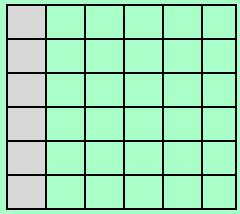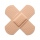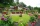# Rectangle - sides 3

If in the rectangle ABCD we enlarge the side a by 5 cm and decrease the side b by 2 cm, the rectangle area will be reduced by 5 cm². When we decrease the length of the side a by 4 cm and and at the same time we increase the length of side b by 3 cm we increases the area of the original rectangle by 5 cm².

Calculate the length of the sides of the rectangle ABCD.

Correct result:

a =  15 cm
b =  7 cm

#### Solution:

(a+5)•(b-2) = a•b - 5
(a-4)•(b+3) = a•b + 5

2a-5b = -5
3a-4b = 17

a = 15
b = 7

Calculated by our linear equations calculator.Our examples were largely sent or created by pupils and students themselves. Therefore, we would be pleased if you could send us any errors you found, spelling mistakes, or rephasing the example. Thank you!

Please write to us with your comment on the math problem or ask something. Thank you for helping each other - students, teachers, parents, and problem authors.Tips to related online calculators
Do you have a system of equations and looking for calculator system of linear equations?

#### You need to know the following knowledge to solve this word math problem:

We encourage you to watch this tutorial video on this math problem:

## Next similar math problems:

• In theIn the rectangle ABCD, the distance of its center from the line AB is 3 cm greater than from the line BC. The circumference of the rectangle is 52 cm. Calculate the contents of the rectangle. Express the result in cm2.
• Area of gardenIf the width of the rectangular garden is decreased by 2 meters and its length is increased by 5 meters, the area of the rectangle will be 0.2 ares larger. If the width and the length of the garden will increase by 3 meters, its original size will increa
• The circumferenceThe circumference and width of the rectangle are in a ratio of 5: 1. its area is 216cm2. What is its length?
• Two patchesPeter taped the wound with two rectangular patches (one over the other to form the letter X). The area sealed with both patches at the same time had a content of 40cm2 and a circumference of 30cm. One of the patches was 8cm wide. What was the width of the
• Rectangular gardenThe perimeter of Peter's rectangular garden is 98 meters. The width of the garden is 60% shorter than its length. Find the dimensions of the rectangular garden in meters. Find the garden area in square meters.
• Rectangle fieldThe field has a shape of a rectangle having a length of 119 m and a width of 19 m. , How many meters have to shorten its length and increase its width to maintain its area and circumference increased by 24 m?
• Rectangular plotThe dimensions of a rectangular plot are (x+1)m and (2x-y)m. If the sum of x and y is 3m and the perimeter of the plot is 36m. Find the area of the diagonal of the plot.
• Side lengthsIn the triangle ABC, the height to the side a is 6cm. The height to side b is equal to 9 cm. Side "a" is 4 cm longer than side "b". Calculate the side lengths a, b.
• An equilateralAn equilateral triangle is inscribed in a square of side 1 unit long so that it has one common vertex with the square. What is the area of the inscribed triangle?
• Rectangular triangleThe lengths of the rectangular triangle sides with a longer leg 12 cm form an arithmetic sequence. What is the area of the triangle?
• CoordinatesDetermine the coordinates of the vertices and the content of the parallelogram, the two sides of which lie on the lines 8x + 3y + 1 = 0, 2x + y-1 = 0 and the diagonal on the line 3x + 2y + 3 = 0
• AP RT triangleThe length of the sides of a right triangle form an arithmetic progression, longer leg is 24 cm long. What are the perimeter and area?
• Right triangle eq2Find the lengths of the sides and the angles in the right triangle. Given area S = 210 and perimeter o = 70.
• FlowerbedWe enlarge the circular flower bed, so its radius increased by 3 m. The substrate consumption per enlarged flower bed was (at the same layer height as before magnification) nine times greater than before. Determine the original flowerbed radius.
• Two gardensThe total area of the two gardens is 864 m2. The first garden is 60 m2 smaller than three times the second garden. What is the area of each garden?
• Isosceles triangleThe perimeter of an isosceles triangle is 112 cm. The length of the arm to the length of the base is at ratio 5:6. Find the triangle area.
• Isosceles trapezoidCalculate the content of an isosceles trapezoid whose bases are at ratio 5:3, the arm is 6cm long and it is 4cm high.